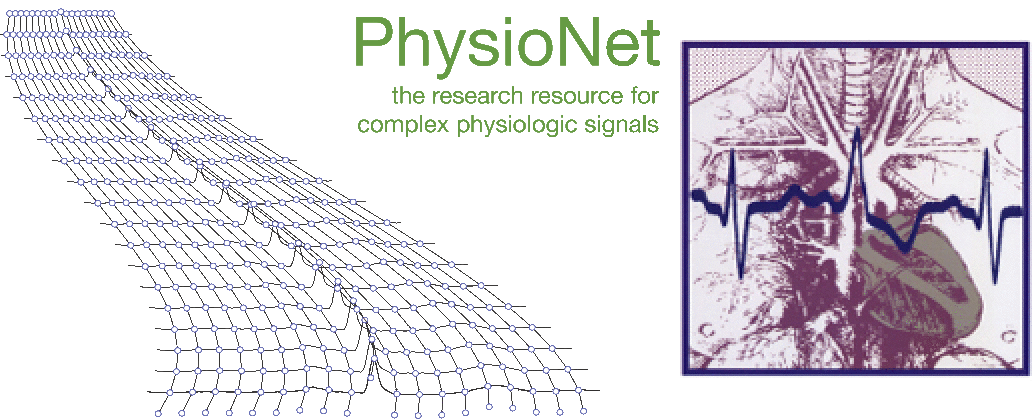# George B. Moody PhysioNet ChallengeSeries 1 is real (from MIT-BIH Normal Sinus Rhythm Database record 16265); series 2 is synthesized using a slightly elaborated version of the example, in which generate looks like this:

float generate(void)
{
float rr;
static float t;
static float omega = 1.0;
static float rrmean = 0.8;

omega += ((float)rand() - RAND_MAX)/(RAND_MAX*100.0) + 0.005;
if (omega < 0.5) omega = 0.5 + ((float)rand())/(RAND_MAX*100.0);
else if (omega > 2.0) omega = 2.0 - ((float)rand())/(RAND_MAX*100.0);
rrmean += ((float)rand() - RAND_MAX)/(RAND_MAX*100.0) + 0.005;
if (rrmean < 0.6) rrmean = 0.6 + ((float)rand())/(RAND_MAX*100.0);
else if (rrmean > 1.1) rrmean = 1.1 - ((float)rand())/(RAND_MAX*100.0);
rr = rrmean + 0.05*sin(omega*t) +
((float)rand() - RAND_MAX)/(RAND_MAX*100.0);
t += rr;
return (rr);
}


Supported by the National Institute of Biomedical Imaging and Bioengineering (NIBIB) under NIH grant number R01EB030362.

Back